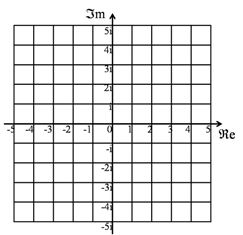The Complex Plane is the two dimensional flat surface (a Euclidean plane) where the coordinates are taken to be the real numbers in one direction, and imaginary numbers in the other. That means that a complex number corresponds to a point on the complex plane.

Also known as the Argand Diagram.AbsoluteValue
ArgandDiagram
CategoryMetaTopic
CauchySequence
CommonCoreDomains
CommonFactor
ComplexConjugate
DifferenceOfTwoSquares
DividingComplexNumbers
Divisor
DomainOfAFunction
FundamentalTheoremOfAlgebra
HolomorphicFunction
ImaginaryNumber
MathematicsTaxonomy
MultiplyingComplexNumbers
Polynomial
Quaternion
RootsOfPolynomials
TypesOfNumber
WhatIsATopic
IsaacNewton
Logarithm
PolarRepresentationOfAComplexNumber
SquareRoot
CosineRule
DiophantineEquation
InverseFunction
MagnitudeOfAVector
NewtonRaphsonMethod
Pythagoras
RiemannHypothesis
Root
RootTwoIsIrrational
ComplexNumber BasinOfAttraction
MagnitudeOfAComplexNumber
NewtonsMethod
PythagorasTheorem
RiemannSurface
RiemannZetaFunction

## You are here

ComplexPlane
ArgandDiagram
Euclid
ImaginaryNumber
RealNumber
CategoryMetaTopic
ComplexConjugate
DividingComplexNumbers
Euler
MultiplyingComplexNumbers
PolarRepresentationOfAComplexNumber
(none) AlgebraicNumber
Axiom
CauchySequence
ContinuedFraction
DedekindCut
Divisor
EuclideanAlgorithm
EuclideanGeometry
FamousPeople
HighestCommonFactor
IrrationalNumber
MersennePrime
PerfectNumber